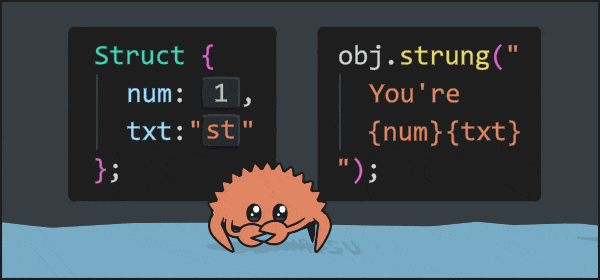## strung

Easy access of struct fields in strings using different/custom pre/postfix: "Hello, {field}"

### 4 releases

 0.1.3 Sep 19, 2022 Sep 14, 2022 Sep 12, 2022 Sep 12, 2022

#45 in Value formatting

Used in 2 crates (via querio)

MIT/Apache

20KB
62 lines🐠 add strung to the dependencies in the `Cargo.toml`:

``````[dependencies]
strung = "0.1.3"
``````

🦀 use/import everything of the prelude in rust:

``````use strung::prelude::*;
``````

🦊 works for unnamed fields:

``````#[derive(Strung)]
struct Struct (&'static str, u32);
fn main(){
let s: String = Struct("Bob", 10).strung("{0} is {1}th");
}
``````

🦊 works for named fields:

``````#[derive(Strung)]
struct Struct {
name: &'static str,
pos: u32,
}
fn main(){
let s: String = Struct{name:"Bob", pos:10}.strung("{name} is {pos}th.");
}
``````

## Prefix and Postix Prefabs

🐎 prefabs are most performant - equal to `strung()`:

``````#[derive(Strung)]
struct Struct (&'static str, u32);
fn main(){
let t = Struct("Bob", 10);
let s = t.strung_curly("{0} is {1}th.");
let s = t.strung_angle("<0> is <1>th.");
let s = t.strung_dollry("\${0} is \${1}th.");
let s = t.strung_dollar("\$0 is \$1th.");
let s = t.strung_hashtag("#0 is #1th.");
}
``````

## Custom Prefix and Postix

🐎 per struct - performance equal to prefabs:

``````#[derive(Strung)]
#[strung("<",">")]
struct Struct (&'static str, u32);
fn main(){
let s: String = Struct("Bob", 10).strung("<0> is <1>th.");
}
``````

🦅 global - easiest, a bit less performant:

``````#[derive(Strung)]
struct Struct (&'static str, u32);
fn main(){
strung::config::static_global("<",">");
let s: String = Struct("Bob", 10).strung_static("<0> is <1>th.");
}
``````

🐍 per call - most flexible:

``````#[derive(Strung)]
struct Struct (&'static str, u32);
fn main(){
let s: String = Struct("Bob", 10).strung_dynamic("<",">","<0> is <1>th.");
}
``````

🦞 currently only for `dollar` and `hashtag` prefab!
🐳 use `#[cscd]`, `#[cascade]`, `#[strung(cscd)]` or `#[strung(cascade)]` on a field:

``````#[derive(Strung)]
struct Struct (&'static str, u32);
#[derive(Strung)]
fn main(){
let s: String = Cascade(11,Struct("Bob", 10))
.strung_dollar("\$1.0 is \$1.1th for the \$0th time!");
}
``````

## Ignore

🦞 if a field type doesn't implement Display, it has to be ignored!
🐎 can also be used to gain minimal amounts of performance!
🐳 use `#[igno]`, `#[strung(igno)]` or `#[strung(ignore)]` on a field
🙉 this example wouldn't compile without `#[igno]`:

``````struct NoDisplay;
#[derive(Strung)]
struct Struct (&'static str, u32, #[igno] NoDisplay);
fn main(){
let s: String = Struct("Bob", 10, NoDisplay)
.strung_dollar("\$0 is \$1th, he won \$2!");
}
``````